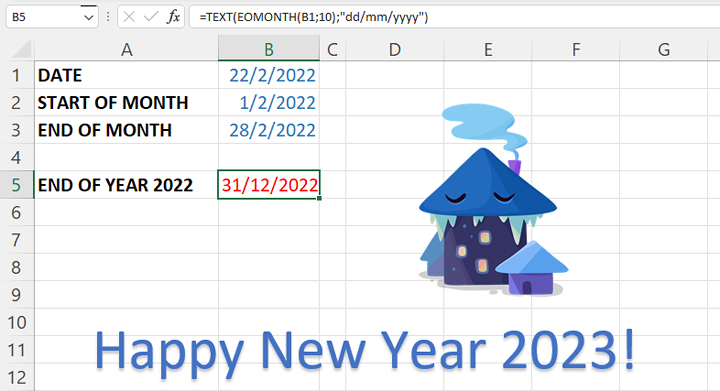# EOMONTH function

Sometimes, while using Excel, you need to specify the date on which a particular month starts or ends in order to perform the desired calculation on that day. Although, with a little effort, these dates can be calculated in the traditional way, you will be greatly relieved by using the EOMONTH function. You will learn more about this function in the following text…

Let’s start from an arbitrary date, e.g. let it be 22/02/2022.

The syntax of the EOMONTH function is:

EOMONTH(<start date>,<number of months>)

The first parameter of the function is the start date, and the second represents the number of months elapsed since the start date. The second parameter can be a negative number, and you will see how it is used a little later.

What is the last day of the current month? Let’s write a formula to calculate this date:

=EOMONTH(B1,0)

How to determine the first day of the month? We count it as the last day of the previous month, increased by 1:

=EOMONTH(B1,-1)+1

Consequently, the first day of the following month is calculated using the formula:

=EOMONTH(B1,0)+1

and the last day using the formula:

=EOMONTH(B1,1)

The EOMONTH function returns an integer as a result. To see the date, you need to format that number as a date, which is most easily achieved by selecting the cell in which you used the EOMONTH function and then using the shortcut CTRL+SHIFT+3.

If you don’t want to spend time on subsequent formatting, you can specify the EOMONTH function within the TEXT function, where you can define how the date will be formatted. For example. if we write the formula:

=TEXT(EOMONTH(B1,0),”dd/mm/yyyy”)

we get the result on 28/02/2022.

So, we the last date in year 2022 is:

=TEXT(EOMONTH(B1,10),”dd/mm/yyyy”)

and if we add 1 to this number, we will get the first date in 2023. Happy New Year!